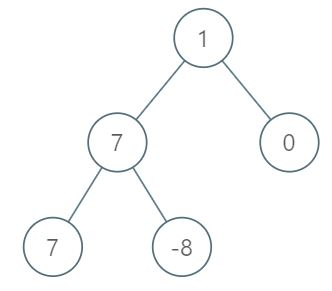Formatted question description: https://leetcode.ca/all/1161.html

# 1161. Maximum Level Sum of a Binary Tree (Medium)

Given the root of a binary tree, the level of its root is 1, the level of its children is 2, and so on.

Return the smallest level X such that the sum of all the values of nodes at level X is maximal.

Example 1:Input: [1,7,0,7,-8,null,null]
Output: 2
Explanation:
Level 1 sum = 1.
Level 2 sum = 7 + 0 = 7.
Level 3 sum = 7 + -8 = -1.
So we return the level with the maximum sum which is level 2.


Note:

1. The number of nodes in the given tree is between 1 and 10^4.
2. -10^5 <= node.val <= 10^5

Companies:

Related Topics:
Graph

## Solution 1. Level-order Traversal

// OJ: https://leetcode.com/problems/maximum-level-sum-of-a-binary-tree/

// Time: O(N)
// Space: O(N)
class Solution {
public:
int maxLevelSum(TreeNode* root) {
if (!root) return 0;
int maxSum = 0, ans = 0, lv = 1;
queue<TreeNode*> q;
q.push(root);
while (q.size()) {
int cnt = q.size(), sum = 0;
while (cnt--) {
auto node = q.front();
q.pop();
sum += node->val;
if (node->left) q.push(node->left);
if (node->right) q.push(node->right);
}
if (sum > maxSum) {
maxSum = sum;
ans = lv;
}
++lv;
}
return ans;
}
};


Java

/**
* Definition for a binary tree node.
* public class TreeNode {
*     int val;
*     TreeNode left;
*     TreeNode right;
*     TreeNode(int x) { val = x; }
* }
*/
class Solution {
public int maxLevelSum(TreeNode root) {
if (root == null)
return 0;
int maxSum = 0;
int maxSumLevel = 0;
int level = 0;
queue.offer(root);
while (!queue.isEmpty()) {
level++;
int curSum = 0;
int size = queue.size();
for (int i = 0; i < size; i++) {
TreeNode node = queue.poll();
curSum += node.val;
TreeNode left = node.left, right = node.right;
if (left != null)
queue.offer(left);
if (right != null)
queue.offer(right);
}
if (curSum > maxSum) {
maxSum = curSum;
maxSumLevel = level;
}
}
return maxSumLevel;
}
}